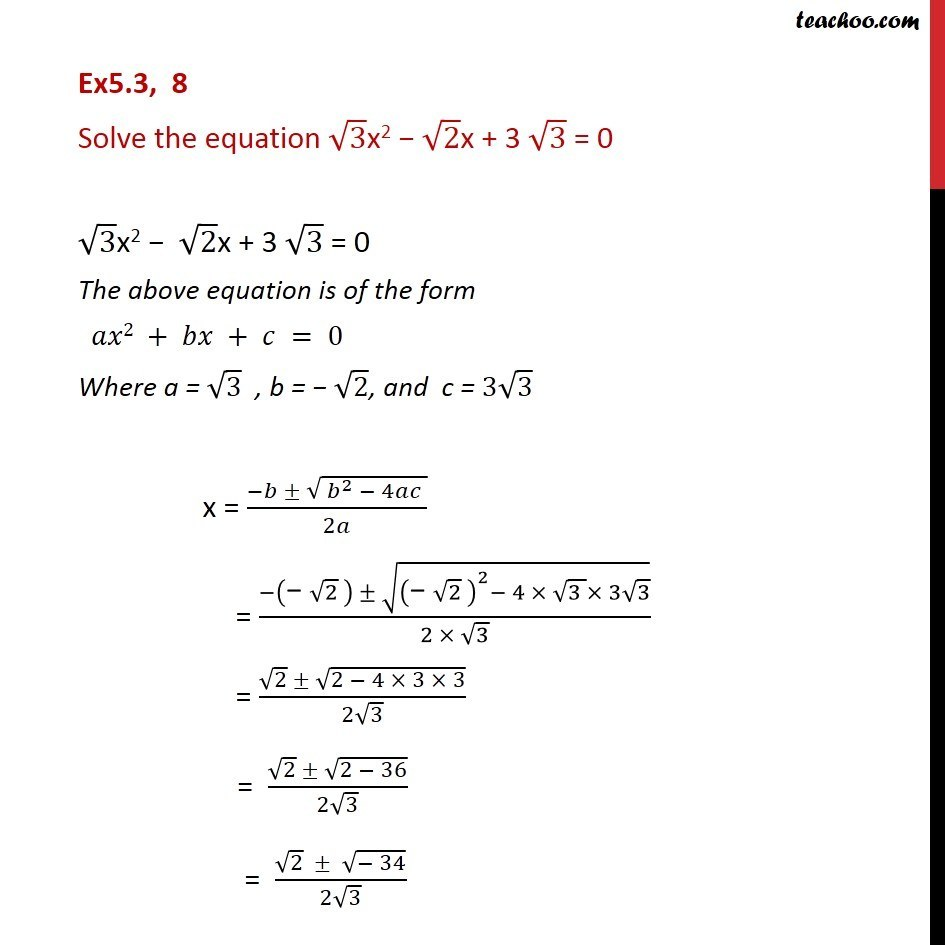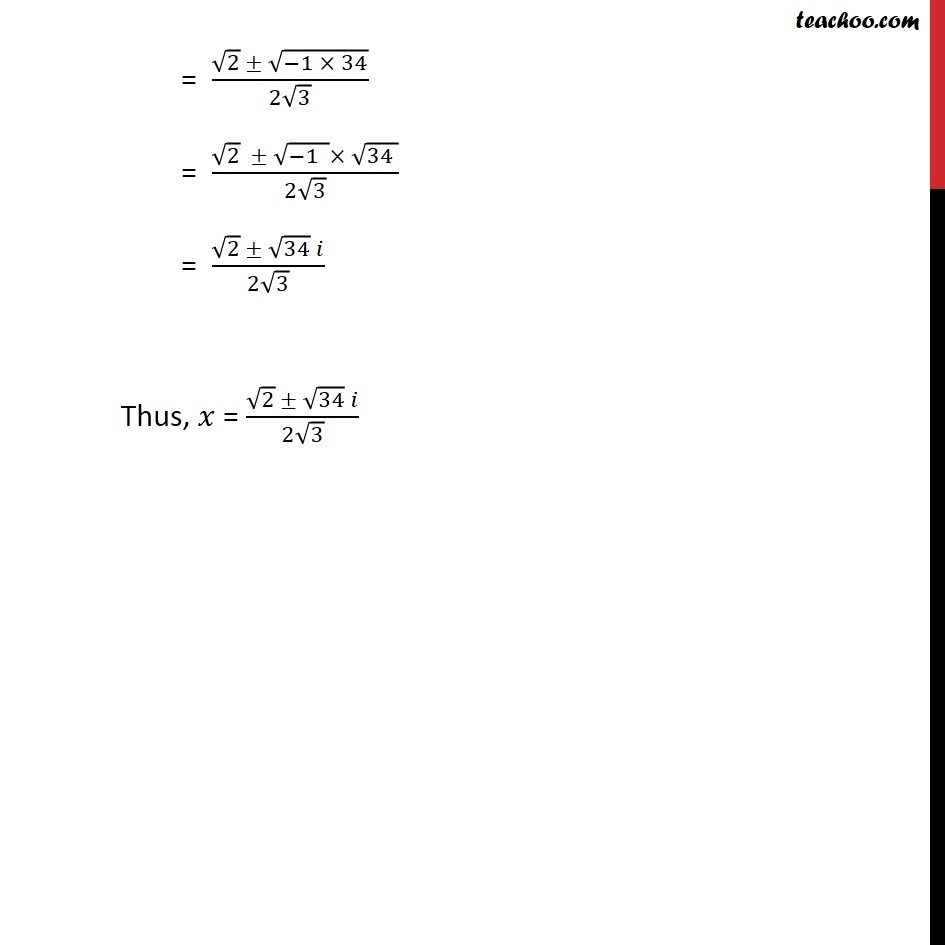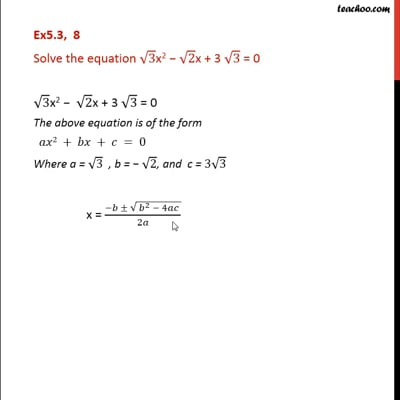Ex 5.3

Chapter 5 Class 11 Complex Numbers
Serial order wiseThis video is only available for Teachoo black users

Get live Maths 1-on-1 Classs - Class 6 to 12

### Transcript

Ex5.3, 8 Solve the equation 3x2 2x + 3 3 = 0 3x2 2x + 3 3 = 0 The above equation is of the form 2 + + = 0 Where a = 3 , b = 2, and c = 3 3 x = ( ( ^2 4 ))/2 = ( (" " 2 ) ((" " 2 )^2 4 (3 ) 3 3) )/(2 3) = ( 2 (2 4 3 3))/(2 3) = ( 2 (2 36))/(2 3) = ( 2 ( 34))/(2 3) = ( 2 ( 1 34))/(2 3) = ( 2 ( 1 ) (34 ))/(2 3) = ( 2 34 )/(2 3) Thus, = ( 2 34 )/(2 3)